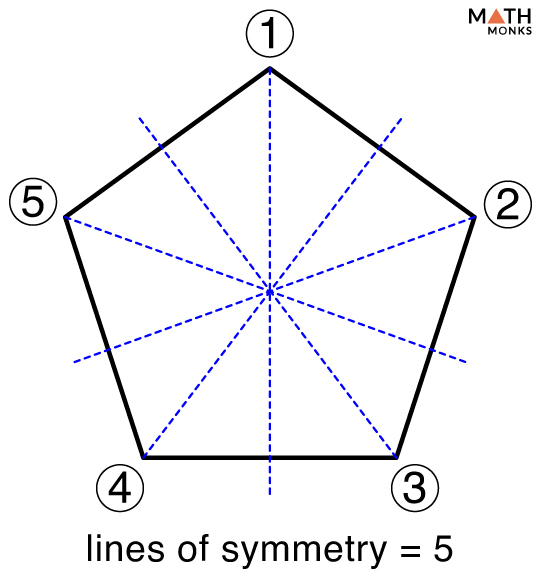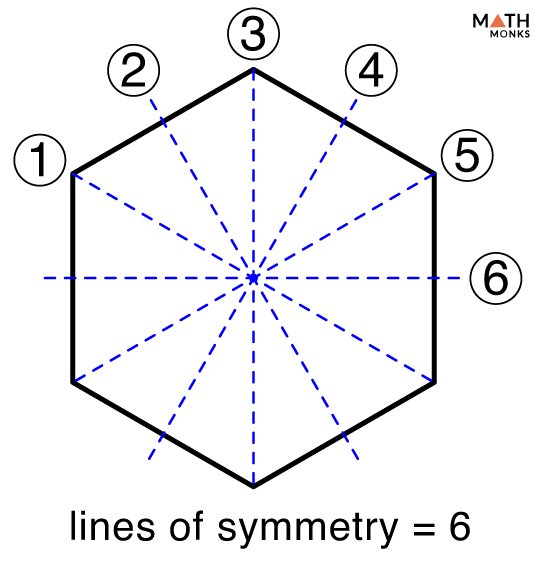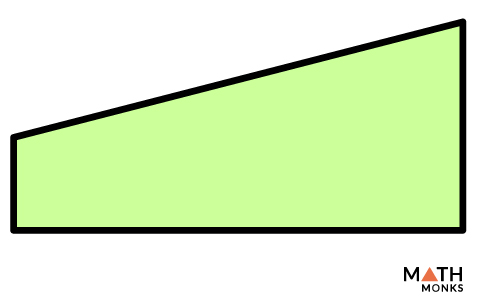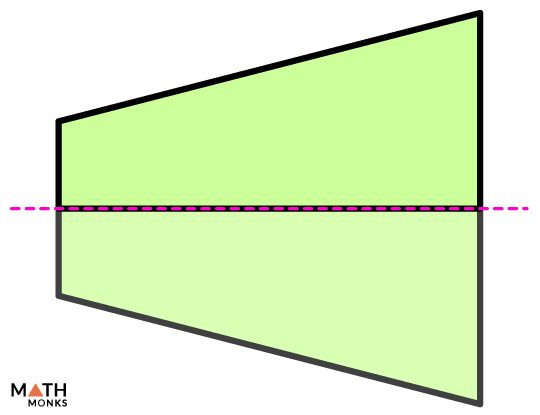# Symmetry

In mathematics, symmetry is the property that divides a geometrical shape into two identical halves. Heart shape, an equilateral triangle, and a rhombus are all examples of symmetry. Such shapes are called symmetric.

### Symmetry in Real Life

We often come across symmetry in nature. For example, the wings of a butterfly, a star fish, feathers of a peacock hives of honeybees, or snowflakes are symmetric by nature. There is also symmetry in the English alphabet.

Several man-made objects also show symmetry like the pyramids of Egypt, the Taj Mahal and several others.

In contrast, any shape that cannot be divided into equal halves is called asymmetric which explains the difference between symmetry and asymmetry.

## Line of Symmetry

See the heart shape, the equilateral triangle or the English alphabets again. There is an imaginary line that divides them into halves. Such a line is called the line of symmetry. Thus, the line of symmetry is the imaginary line that divides an object into two identical halves. It is also called the axis of symmetry.

The halves are exact mirror images. Thus, when we fold the shape along a line of symmetry, the halves overlap entirely with one another.

A shape can have one, two, or more, or even an infinite line of symmetry.

In mathematics, lines of symmetry are of three types: 1) vertical Line of Symmetry, 2) Horizontal Line of Symmetry, and Diagonal Line of Symmetry.

## Types of Symmetry

We can observe symmetry in a shape when we turn, flip, or slide it. Accordingly, there are following types of symmetry:

### Translational Symmetry

A shape shows translational symmetry when they are moved from one position to another with the same orientation in the forward and backward motion. It is sliding of an object about an axis.

### Rotational Symmetry

A shape shows rotational symmetry when we rotate it around a central point at an angle other than 360°, and the outcome is the same as the shape’s original appearance.

### Reflectional Symmetry

A shape has a reflectional (reflective) symmetry if the line of symmetry divides the object into two equal halves such that each half is a mirror image of the other. It is thus nothing but line symmetry we studied earlier.

### Glide Symmetry

It is the combination of both translation and reflection symmetry. We can alter the combination; reflection at first and translation after it, or vice-versa.

### Point Symmetry

A shape has point symmetry when every part of an object has a matching part in another object but in the opposite direction.

## Solved ExamplesHow many lines of symmetry does a pentagon have?

Solution:A pentagon has 5 lines of symmetry.How many lines of symmetry does a hexagon have?

Solution:A hexagon has 6 lines of symmetryHow many lines of symmetry does the given shape have? What line of symmetry does it show?

Solution:The given shape has one line of symmetry.
It has a horizontal line of symmetry.

## FAQs

Which letter has rotational symmetry?

Ans. Letters such as I, J, K, and L have rotational symmetry.

• More Resources